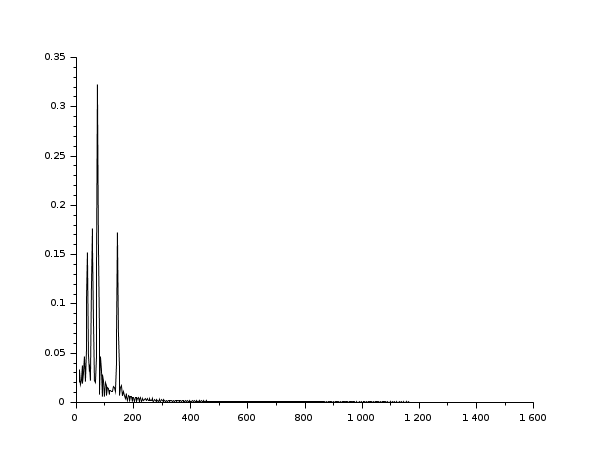# analyze

frequency plot of a sound signal

### Arguments

fmin,fmax,rate,points

scalars. default values fmin=100,fmax=1500,rate=22050,points=8192;

### Description

Make a frequency plot of the signal `w` with sampling rate `rate`. The data must be at least `points` long. The maximal frequency plotted will be `fmax`, the minimal `fmin`.

### Examples

```// At first we create a time vector of 0.5 seconds sampled at 22050 Hz:
t = 0 : 1/22050 : 0.5*(1-%eps);

// Then we generate the sound.
s=sin(440*t)+sin(220*t)/2+sin(880*t)/2;
[nr,nc]=size(t);
s(nc/2:nc)=sin(330*t(nc/2:nc));
analyze(s);```Report an issue << Sound file handling Sound file handling auread >>